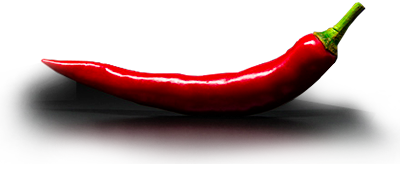# GROWERS GUIDE

## Plant nutrient labelling

All governments enforce detailed guidelines on the format and type of information that must be declared on the labels of horticultural products.

## How to interpret the label analyses of water based nutrients

With the common exception of potassium and phosphorus, most nutrient labels state the concentration of nutrient elements in their elemental form – as per the “Element” column in Table 7.20.

K2O and P2O5: By convention, many labels represent potassium (K) as dipotassium oxide (K2O) - see Fig 7.4. To convert K2O to K, multiply the K2O figure by 0.83. Similarly, phosphorus (P) is often represented as phosphorus pentoxide (P2O5). To convert P2O5 to P, multiply the P2O5 figure by 0.44.

Concentration: The concentration of elements is represented as percentage (%). Specifically this is % m/v i.e. percentage mass per unit of volume. Multiplying this figure by 10 expresses the concentration in grams per litre e.g. 3.0% equals 30g/L. Alternatively, multiplication by 10,000 expresses the concentration in ppm or mg/L. For example, 3.0% equals 30,000ppm.

## How to compare nutrient label analyses of 1, 2 & 3-part labels

Label analysis figures for 2 & 3-part nutrients are usually based upon the concentration of nutrients in the individual packs. Therefore, in order to make a meaningful comparison between a 2-part and 1-part nutrient, it is necessary to first double the figures quoted on the 1-part. This ensures the contents of equal volumes are being compared. For example, 2 x 1 litre bottles of the 1-part with 2 x 1 litre bottles of the 2-part (‘A’ and ‘B’) .

● To compare a 1-part with a 3-part, first ‘triple’ the 1-part figures.
● To compare a 2-part with a 3-part, first halve the 2-part figures before multiplying them by 3.

This information makes it possible to compare analyses. Graph 7.30, displays the results of a meaningful comparison between 1, 2 and 3-part nutrients.

## Nutrient element concentration in the working nutrient solution yielded by a specific dose

To determine the concentration of individual elements in the working nutrient solution, the individual analysis specification should first be converted into ppm (or mg/L), then multiplied by the dose rate (per litre). For example, if part ‘B’ of a 2-part contains 3.0% phosphate as P2O5, then when this part is used at 4ml per litre, it will yield 53ppm phosphorus as P. That is:

Step 1. “3.0% P2O5” = 30,000ppm as P2O5 (i.e. 3.0% x 10,000).

Step 2. Convert this figure to P: 30,000ppm as P2O5 = 13,200ppm as P (i.e. 30,000ppm x 0.44).

Step 3. 4ml per litre yields 53ppm P (i.e. 13,200ppm x 4ml ÷ 1,000ml).

[Growers Guide Main Index]